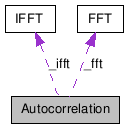# Autocorrelation Class Reference

Algorithm to perform an autocorrelation of vectors of Real values. More...

`#include <Autocorrelation.h>`

Collaboration diagram for Autocorrelation:[legend]

List of all members.

## Public Member Functions

Autocorrelation (int inputSize, int maxLag, int minLag, bool useFft)
~Autocorrelation ()
void process (const MatrixXR &frames, MatrixXR *autocorrelation)
int inputSize () const
void setInputSize (int size, bool callSetup=true)
int minLag () const
void setMinLag (int lag, bool callSetup=true)
int maxLag () const
void setMaxLag (int lag, bool callSetup=true)
bool useFft () const
void setUseFft (bool useFft, bool callSetup=true)

## Detailed Description

Algorithm to perform an autocorrelation of vectors of Real values.

This class represents an object to perform a correlation of a vector with itself.

The correlation can be performed using two methods:

1. Direct method
2. FFT method

The Direct method consists in applying the correlation formula directly in the time domain.

The FFT method consists in performing an Fast Fourier Transform of the vector and multiply it by its conjugate. Finally the algorithm applies an IFFT to the result of the multiplication in order to obtain the autocorrelation for all the time lags.

The Direct method performs faster than the FFT method only on vectors of small sizes. The decision point for selecting one of the two methods depends on the platform.

The method performed can be specified using setUseFft().

Correlation, PitchACF

## Constructor & Destructor Documentation

 Autocorrelation ( int inputSize, int maxLag, int minLag, bool useFft )

Constructs an Autocorrelation object with the specified inputSize, maxLag and minLag settings.

Parameters:
 inputSize size of the inputs arrays to be autocorrelated, must be > 0. The algorithm performs faster for sizes which are a power of 2. maxLag maximum lag to be calculated minLag minimum lag to be calculated useFft determines whether or not to use the FFT method

References setInputSize(), setMaxLag(), setMinLag(), and setUseFft().

 ~Autocorrelation ( )

Destroys the Autocorrelation algorithm and frees its resources.

## Member Function Documentation

 void process ( const MatrixXR & frames, MatrixXR * autocorrelation )

Performs an autocorrelation on each of the rows of frames. Puts the resulting autocorrelations in the rows of autocorrelation.

Parameters:
 frames matrix of Real values. The number of columns of frames must be equal to the inputSize property. autocorrelation pointer to a matrix of Real values for the output. The matrix should have the same number of rows as frames and maxLag - minLag columns.
Note that if the output matrix is not of the required size it will be resized, reallocating a new memory space if necessary.

References IFFT::process(), and FFT::process().

Referenced by PitchACF::process(), and LPC::process().

 int inputSize ( ) const

Returns the size of the input arrays to be autocorrelated. The default is 1024.

setInputSize()

 void setInputSize ( int size, bool callSetup = `true` )

Specifies the size of the input. The given size must be higher than 0. Note that if size is a power of 2 the algorithm will perform faster.

inputSize()

Referenced by Autocorrelation().

 int minLag ( ) const

Returns the minimum lag to be calculated. The default is 0.

maxLag(), setMinLag(), setMaxLag()

 void setMinLag ( int lag, bool callSetup = `true` )

Specifies the minimum lag of the autocorrelation. The given lag will be constratined between -inputSize + 1 and inputSize.

minLag(), maxLag(), setMaxLag()

Referenced by Autocorrelation().

 int maxLag ( ) const

Returns the maximum lag to be calculated. The default is inputSize.

minLag(), setMinLag(), setMaxLag()

 void setMaxLag ( int lag, bool callSetup = `true` )

Specifies the maximum lag of the autocorrelation. The given lag will be constratined between -inputSize + 1 and inputSize.

minLag(), maxLag(), setMinLag()

Referenced by Autocorrelation().

 bool useFft ( ) const

Returns `true` if the FFT method should be used for the autocorrelation. The default is True for inputSize larger than 128; otherwise it is False.

setUseFft()

 void setUseFft ( bool useFft, bool callSetup = `true` )

Specifies whether the autocorrelation should be performed using the FFT method.

Generated on Tue Mar 31 20:38:32 2009 for Loudia by1.5.6